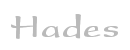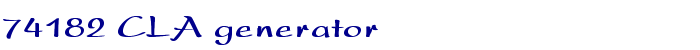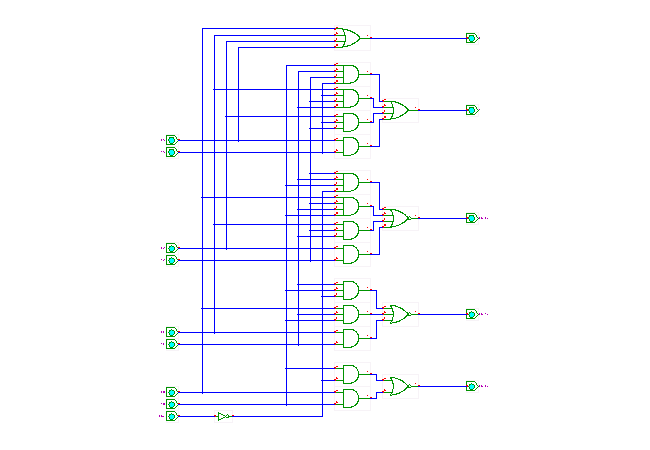TAMS / Java / Hades / applets (print version): contents | previous | next

#### TTL-series 74182 CLA generator circuitDescription

This applet shows the internal structure of the 74182 4-bit Carry-Lookahead Generator.

Click the input switches or type the following bindkeys: 'c' for CN/ (active-low carry in), and '1'..'4' for 'X0'..'X3', and '5'..'8' for 'Y0'..'Y3'.

The output signals are calculated in two-level logic as:

```  X = X3 + X2 + X1 + X0
Y = Y3 (X3 + Y2) (X3 + X2 + Y1) (X3 + X2 + X1 + Y0)

/Cn+x = /(Y0 (X0 + Cn))
/Cn+y = /(Y1 (X1 + Y0 (X0 + Cn)))
/Cn+z = /(Y2 (X2 + Y1 (X1 + Y0 (X0 + Cn))))
```

Run the applet | Run the editor (via Webstart)

Impressum | 24.11.06